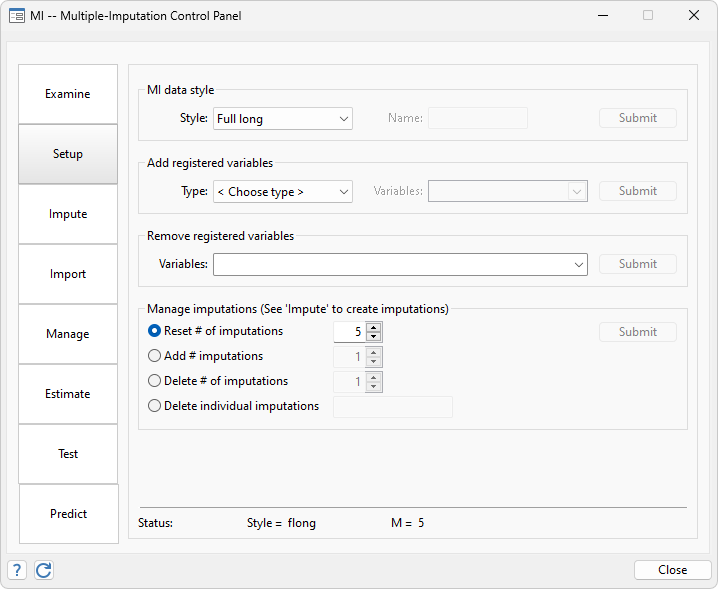Account for missing data in your sample using multiple imputation. Choose from univariate and multivariate methods to impute missing values in continuous, censored, truncated, binary, ordinal, categorical, and count variables. Then, in a single step, estimate parameters using the imputed datasets, and combine results. Fit a linear model, logit model, Poisson model, multilevel model, survival model, or one of the many other supported models. Use the mi command, or let the Control Panel interface guide you through your entire MI analysis.

IMPUTATION METHODS

• Multivariate normal
• Chained equations
• Linear regression
• Predictive mean matching
• Truncated regression
• Interval regression
• Logistic
• Ordered logit
• Multinomial (polytomous) logit
• Poisson
• Negative binomial
• User-defined

DATA MANAGEMENT

• Tabulate missing values
• Create summary variables of missing-value patterns
• Identify varying and super-varying variables
• Execute commands across imputations
• Export and import foreign data
• Create functions of imputed variables

ESTIMATION AND INFERENCE

• Automatically pool results from each dataset
• Joint tests of coefficients
• Linearly and nonlinearly transformed coefficients
• Linear and nonlinear MI predictions

POSTESTIMATION SELECTOR

• View and run all postestimation features for your command
• Automatically updated as estimation commands are run

UTILITIES

• Change style of multiple-imputation datasets
• Extract datasets
• Verify and repair consistency of data

• Introduction to mi
• Introduction to multiple-imputation analysis

CONTROL PANEL

• Guides you along from start to finish
• Set up data and impute missing values or import data
• Perform data management
• Perform estimation and inference
• Command log produced to ensure reproducibilityWatch handling missing data in Stata tutorials